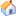Teaching

Current Academic Year 2017/2018

 Semester Courses Course Website 171

Previous Semesters

 Semester Courses Course Website 092 092 091 Math 260: Introduction to DE & Linear Algebra  (Grades) 082 081 Math 550: Linear Algebra 081 Math 260: Introduction to DE & Linear Algebra 072 Math 202: Elements of Differential Equations071 Math 550: Linear Algebra071 Math 355: Linear Algebra071 Math 260: Introduction to DE & Linear Algebra062 Math 202: Elements of Differential Equations061 On Sabbatical Leave 052 On Leave 051 Math 101: Calculus I042 Math 102: Calculus II041 Math 311: Advanced Calculus041 Math 101: Calculus I032 Math 260: Introduction to DE & Linear Algebra031 Math 202: Elements of Differential EquationCourses Taught

 Algebra and Trigonometry (Math 001, Math 002) Calculus I (Math 101) Calculus II (Math 102) Calculus III (Math 201) Finite Mathematics (Math 131) Differential Equations (Math 202) Introduction to Sets and Structures (Math 232) Introduction to Differential Equations & Linear Algebra (Math 260) Introduction to Linear Algebra (Math 280) Advanced Calculus (Real Analysis) (Math 311) Modern Algebra I (Math 345) Linear Algebra (Math 355) Modern Algebra II (Math 450) Algebra I (Math 552) Graduate Course
 Linear Algebra (Math 550) Graduate Course

Course Coordination

• The coordinator of Math 131 (Finite Mathematics) in 2001.

• Coordinator for the final exam for some Math courses.

• The coordinator of Math 260 (Differential Equations and Linear Algebra) in 2008.

• The coordinator of Math 202 (Differential Equations) in 2009.

Course development

• Being a committee chairman, I contributed towards the design of Math 499, “Summer Training Program for Math undergraduates” in 2002.

• I contributed in the revision of BS Math program in 2002.

• I contributed in writing the course description for Math courses101, 102 and 201 in 2000, and also revising the content of the Math courses 232 and 345 in 2001.

• I was involved in preparation and translation of supplementary material for the preparatory year Math in 2000.

Teaching Projects

“Student’s Learning Process in Pre-Calculus and Calculus Courses at KFUPM; Identification of Problems and Possible Remedies”. (Completed in 2006)

Thesis supervision

“A study of Special Mappings on Prime Rings”, MS Thesis, completed in 2008.